# 5. (20 pts) Consider the following string: char oscarwilde = "I can resist everything except temptation"...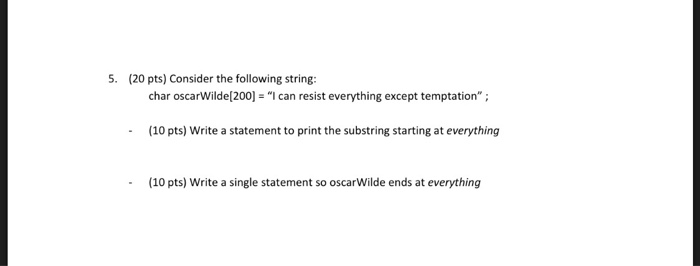5. (20 pts) Consider the following string: char oscarwilde = "I can resist everything except temptation" ; (10 pts) Write a statement to print the substring starting at everything (10 pts) Write a single statement so oscarWilde ends at everything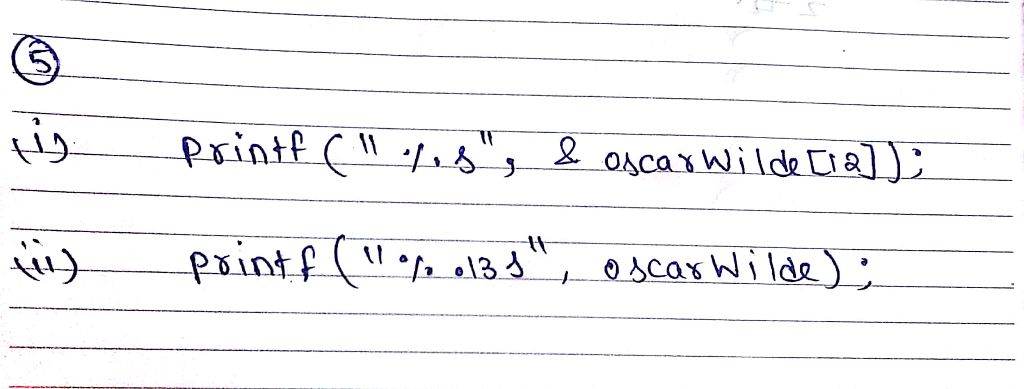#### Earn Coin

Coins can be redeemed for fabulous gifts.

Similar Homework Help Questions
• ### Given the following classes: StringTools.java: public class StringTools { public static String reverse(String s){ char[] original=s.toCharArray();...

Given the following classes: StringTools.java: public class StringTools { public static String reverse(String s){ char[] original=s.toCharArray(); char[] reverse = new char[original.length]; for(int i =0; i<s.length(); i++){ reverse[i] = original[original.length-1-i]; } return new String(reverse); } /**  * Takes in a string containing a first, middle and last name in that order.  * For example Amith Mamidi Reddy to A. M. Reddy.  * If there are not three words in the string then the method will return null  * @param name in...

• ### C language 5. (14 pts) Write a single statement to do each of the following: a....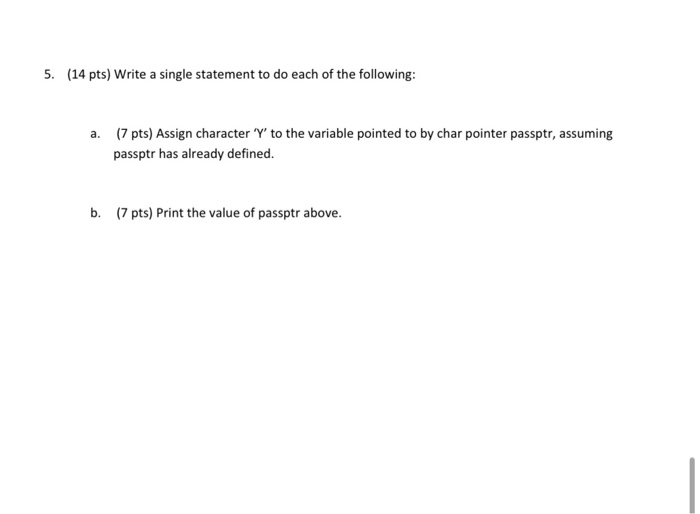C language 5. (14 pts) Write a single statement to do each of the following: a. (7 pts) Assign character 'Y' to the variable pointed to by char pointer passptr, assuming passptr has already defined. b. (7 pts) Print the value of passptr above.

• ### Question 6 Predict the output of the following question: 8 pts #include <stdio.h> #include<string.h> char al(5)={...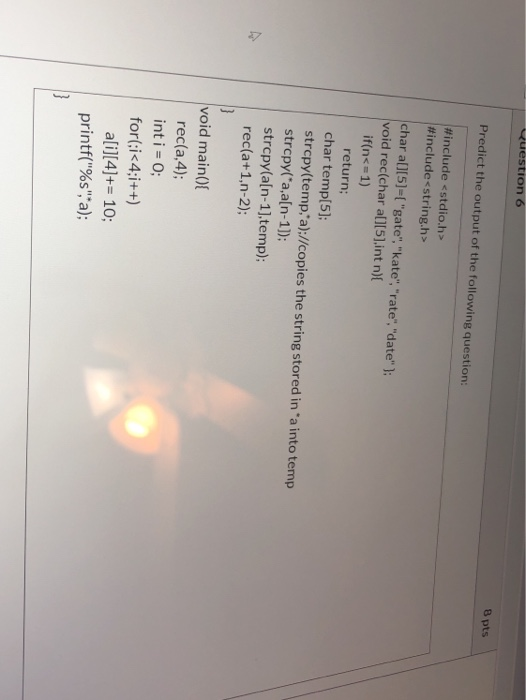Question 6 Predict the output of the following question: 8 pts #include <stdio.h> #include<string.h> char al(5)={ "gate", "kate","rate","date" ); void rec(char a[], int n){ if(n<= 1) return; char temp; strcpy(temp'a);//copies the string stored in a into temp strcpy(*a,a[n-1]); strcpy(a[n-1),temp); rec(a+1,n-2); } void main() { rec(a 4); int i = 0; for(;i<4;i++) a[i]+= 10; printf("%s", a); }

• ### (15 pts) Using the following example, int a, p=&a; *p = 20; Give the two major...

(15 pts) Using the following example, int a, p=&a; *p = 20; Give the two major differences of * and & in the above example. (35 pts) Do the following in a program segment step by step. The next step uses the results of the previous step and earlier, using a single statement only and none for manual work. Declare an array called a of float of size 10 and initialize it to 2.5, -3.3, 5.9, 1.0, 3.5, 4.3, -7.3,...

• ### 5. (12 points) Consider the following code fragment (part of a program): int index 3; String...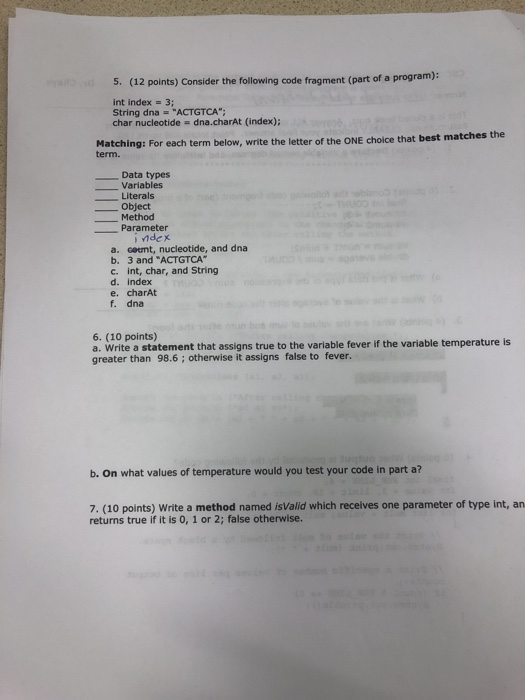5. (12 points) Consider the following code fragment (part of a program): int index 3; String dna "ACTGTCA char nucleotide dna.charAt (index); Matching: For each term below, write the letter of the ONE choice that best matches the term. Data types Variables Literals Object Method ーParameter nde x a. coumt, nucleotide, and d b. 3 and "ACTGTCA c. int, char, and String d. index e. charAt f. dna na 6. (10 points) a. Wrte a statement that assigns true to...

• ### Please use Python def findSubstrings(s):     # Write your code here Consider a string, s = "abc"....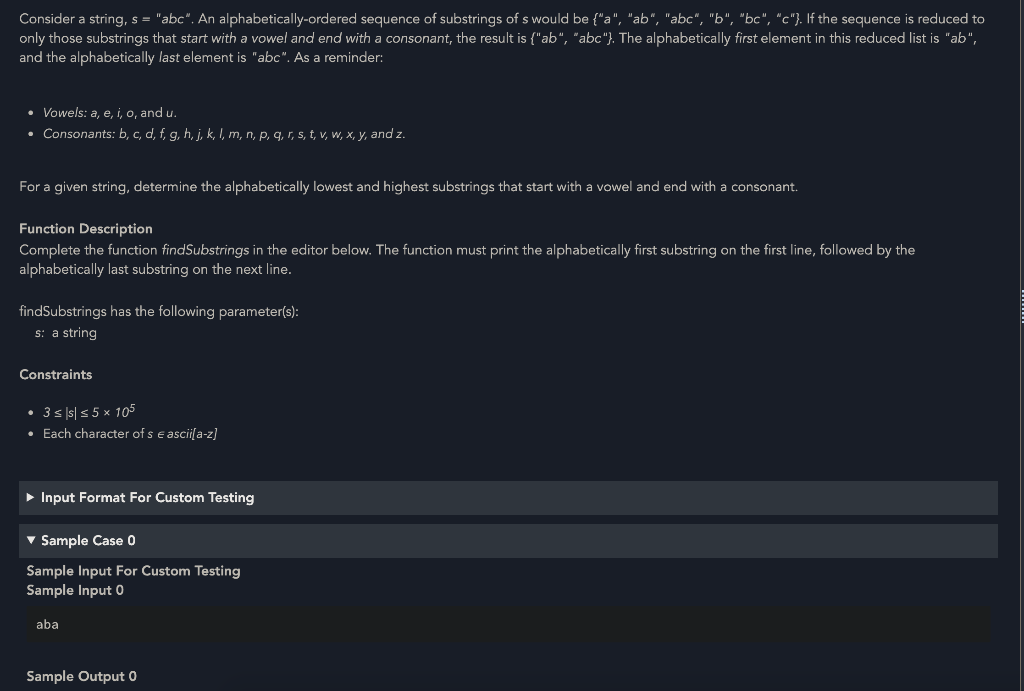Please use Python def findSubstrings(s):     # Write your code here Consider a string, s = "abc". An alphabetically-ordered sequence of substrings of s would be {"a", "ab", "abc", "b", "bc", "c"}. If the sequence is reduced to only those substrings that start with a vowel and end with a consonant, the result is {"ab", "abc"}. The alphabetically first element in this reduced list is "ab", and the alphabetically last element is "abc". As a reminder: • Vowels: a, e, i,...

• ### Problem 4. (20 points) Consider the following C structs: struct S1 { char a; char b;...

Problem 4. (20 points) Consider the following C structs: struct S1 { char a; char b; char c; } struct S2 { S1 d; S1 e; S1 *f; short g; char h; double i; int j; } A. Show how the S1 struct would be in memory on a 64-bit machine. Label the bytes that belong to the various fields with their names and clearly mark the end of the struct. Use the character 'X' to indicate bytes that are...

• ### this for my class SQL language Question 3 10 pts What errors are in the following...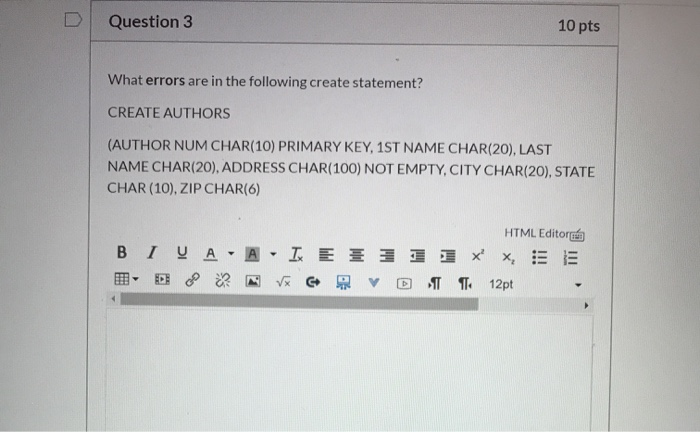this for my class SQL language Question 3 10 pts What errors are in the following create statement? CREATE AUTHORS (AUTHOR NUM CHAR(10) PRIMARY KEY, 1ST NAME CHAR(20), LAST NAME CHAR(20), ADDRESS CHAR(100) NOT EMPTY.CITY CHAR(20), STATE CHAR(10), ZIP CHAR(6) HTML Editora B I VA -A I ET 1 1 1 XX, SE - E o N V PRVO T 12pt Question 4 10 pts Write the SQL to add the following customer to the Colonial Adventure Tours The customer...

• ### C program flow char Make flow-charts to do the following. Each flowchart element can only pertora...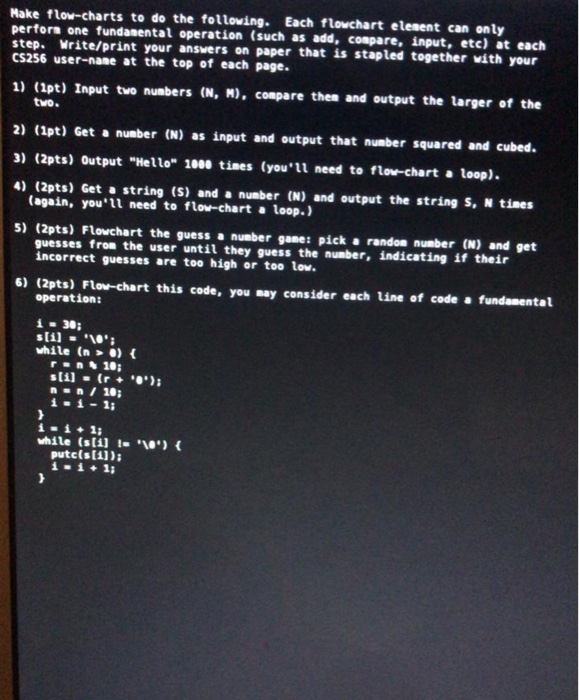C program flow char Make flow-charts to do the following. Each flowchart element can only pertora one fundamental operation (such as add, compare, input, etc) at each step. Write/print your answers on paper that is stapled together with your CS256 user-nane at the top of each page. 1) (1pt) Input two numbers (N, N), compare then and output the larger of the tvo. 2) (1pt) Get a number (N) as input and output that number squared and cubed. 3) (2pts)...

• ### code: 3. (20 pts.) Consider the following fragment of for (i-0; i<=100; i-i++) { a [i]...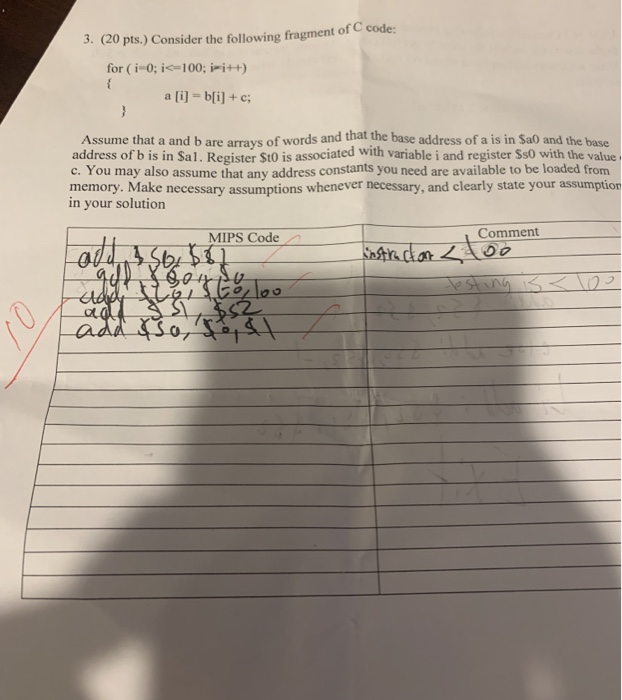code: 3. (20 pts.) Consider the following fragment of for (i-0; i<=100; i-i++) { a [i] = b[i] + c; Assume that a and b are arrays of words and that the base address of a is in Sao and the base address of b is in Sal. Register St0 is associated with variable i and register \$so with the value c. You may also assume that any address constants you need are available to be loaded from memory. Make...

Free Homework App